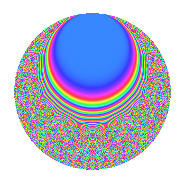# Properties

 Label 43.10.cLevel 43 Weight 10 Character orbit c Rep. character $$\chi_{43}(6,\cdot)$$ Character field $$\Q(\zeta_{3})$$ Dimension 64 Newform subspaces 1 Sturm bound 36 Trace bound 0

# Related objects

## Defining parameters

 Level: $$N$$ $$=$$ $$43$$ Weight: $$k$$ $$=$$ $$10$$ Character orbit: $$[\chi]$$ $$=$$ 43.c (of order $$3$$ and degree $$2$$) Character conductor: $$\operatorname{cond}(\chi)$$ $$=$$ $$43$$ Character field: $$\Q(\zeta_{3})$$ Newform subspaces: $$1$$ Sturm bound: $$36$$ Trace bound: $$0$$

## Dimensions

The following table gives the dimensions of various subspaces of $$M_{10}(43, [\chi])$$.

Total New Old
Modular forms 68 68 0
Cusp forms 64 64 0
Eisenstein series 4 4 0

## Trace form

 $$64q - 70q^{2} + 307q^{3} + 16038q^{4} + 681q^{5} - 1281q^{6} + 957q^{7} - 10920q^{8} - 255339q^{9} + O(q^{10})$$ $$64q - 70q^{2} + 307q^{3} + 16038q^{4} + 681q^{5} - 1281q^{6} + 957q^{7} - 10920q^{8} - 255339q^{9} - 6237q^{10} - 180682q^{11} + 274344q^{12} + 115245q^{13} - 171208q^{14} + 138833q^{15} + 4289206q^{16} + 65180q^{17} + 603985q^{18} + 39150q^{19} - 2709775q^{20} - 3105162q^{21} + 598456q^{22} + 1285907q^{23} - 292550q^{24} - 14709917q^{25} + 5332695q^{26} - 20118002q^{27} - 5475298q^{28} + 1274091q^{29} + 28424751q^{30} + 2899781q^{31} - 16322030q^{32} + 30668912q^{33} + 6822099q^{34} - 14750922q^{35} - 113819232q^{36} - 13772217q^{37} + 2700532q^{38} + 49876982q^{39} - 55802359q^{40} + 5375394q^{41} - 62768266q^{42} - 4250879q^{43} - 125171958q^{44} + 74719384q^{45} - 91405220q^{46} + 46104596q^{47} + 294588191q^{48} - 233221733q^{49} + 380339342q^{50} + 229598406q^{51} + 166281612q^{52} + 125396641q^{53} - 137752484q^{54} + 139471854q^{55} + 41104589q^{56} - 289586721q^{57} - 183782516q^{58} - 637949078q^{59} - 70735747q^{60} - 95573327q^{61} + 181277053q^{62} + 226632970q^{63} + 712599660q^{64} - 498753718q^{65} - 349240445q^{66} - 276270786q^{67} + 1034771928q^{68} - 199705103q^{69} + 1780847990q^{70} + 651945247q^{71} - 270063054q^{72} - 182725322q^{73} - 193737672q^{74} - 3155252572q^{75} + 492133584q^{76} + 216024528q^{77} + 2562586420q^{78} - 376932221q^{79} - 783129021q^{80} - 2146956496q^{81} + 2373824428q^{82} + 1008544071q^{83} - 9413139208q^{84} - 1131353054q^{85} - 1641459050q^{86} + 5060470018q^{87} + 369936980q^{88} + 2244184366q^{89} + 3302428348q^{90} + 3951711q^{91} + 4361095637q^{92} + 3133616089q^{93} + 3002217406q^{94} + 1515822931q^{95} + 69408612q^{96} - 6951085934q^{97} + 1120193981q^{98} + 1264470179q^{99} + O(q^{100})$$

## Decomposition of $$S_{10}^{\mathrm{new}}(43, [\chi])$$ into newform subspaces

Label Dim. $$A$$ Field CM Traces $q$-expansion
$$a_2$$ $$a_3$$ $$a_5$$ $$a_7$$
43.10.c.a $$64$$ $$22.147$$ None $$-70$$ $$307$$ $$681$$ $$957$$

## Hecke characteristic polynomials

There are no characteristic polynomials of Hecke operators in the database